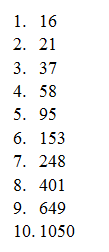# Mathsmagic : Multiplication and Addition Doing Fibonacci’s Lightning Calculation

On a piece of paper, write the number 1 to 10 in a column. You are now all set to amaze with the speed at which you can add ten numbers.

Ask your friend to choose any two two-digit numbers and write the numbers down in the first two spaces of your column, one under the other. Your friend then makes a third number by adding these first two numbers together and writes it below the first two, in effect starting a chain of numbers. They make a fourth number by adding the second and third, a fifth by adding the third and fourth, and so on, until your column of ten numbers is full.

To show how brilliant you are, you can turn away once your friend has understood the idea, say after the seventh number in the list. Now you can’t even see the numbers being written.
Meanwhile, with your back turned, you are actually multiplying that seventh number by 11 to get the final answer.

Let’s imagine your friend chose 16 and 21 to start with. The list would look like this:You now turn round and write the sum of all ten numbers straight away! Lightning quick, you say it is 2728. Let them do it slowly on a calculator to show your brilliant mind skills are 100 per cent correct. The final answer just involves multiplying the seventh number by 11. Why?

Well this chain of numbers where the next term is made by adding the previous two terms is called a Fibonacci sequence. Fibonacci sequences have special mathematical properties that most folk don’t know about…

So let’s look at the trick. We start with the two numbers A and B. The next number is A+B, the next number is B added to A+B which is A+2B and so on. Going through the number chain we find:

Adding up all 10 numbers in the chain gives us a grand total of 55A+88B – check it yourself. But look at the seventh number in your column… this line is 5A+8B. It is exactly the total of the chain but divided by 11!

So working backwards, you can get the final total by multiplying the seventh term by 11. And the maths proves this lightning calculation will work for any two starting values A and B.

It is up to you to present this trick in such a way that it looks like you are just very, very clever. Which of course you are, as you now know how to use a Fibonacci sequence for magic.

## 1 comment for "Mathsmagic : Multiplication and Addition Doing Fibonacci’s Lightning Calculation"

1.